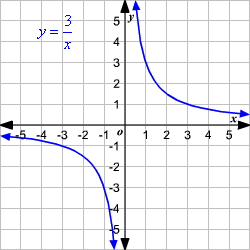# Inverse Variation

While direct variation describes a linear relationship between two variables , inverse variation describes another kind of relationship.

For two quantities with inverse variation, as one quantity increases, the other quantity decreases.

For example, when you travel to a particular location, as your speed increases, the time it takes to arrive at that location decreases. When you decrease your speed, the time it takes to arrive at that location increases. So, the quantities are inversely proportional.

An inverse variation can be represented by the equation $xy=k$ or $y=\frac{k}{x}$ .

That is, $y$ varies inversely as $x$ if there is some nonzero constant $k$ such that, $xy=k$ or $y=\frac{k}{x}$ where $x\ne 0,y\ne 0$ .

Suppose $y$ varies inversely as $x$ such that $xy=3$ or $y=\frac{3}{x}$ . That graph of this equation shown.Since $k$ is a positive value, as the values of $x$ increase, the values of $y$ decrease.

Note: For direct variation equations, you say that $y$ varies directly as $x$ . For inverse variation equations, you say that $y$ varies inversely as $x$ .

### Product Rule for Inverse Variation

If $\left({x}_{1},{y}_{1}\right)$ and $\left({x}_{2},{y}_{2}\right)$ are solutions of an inverse variation, then ${x}_{1}{y}_{1}=k$ and ${x}_{2}{y}_{2}=k$ .

Substitute ${x}_{1}{y}_{1}$ for $k$ .

${x}_{1}{y}_{1}={x}_{2}{y}_{2}$ or $\frac{{x}_{1}}{{x}_{2}}=\frac{{y}_{2}}{{y}_{1}}$

The equation ${x}_{1}{y}_{1}={x}_{2}{y}_{2}$ is called the product rule for inverse variations.

Example:

In a factory, $10$ men can do the job in $30$ days. How many days it will take if $20$ men do the same job?

Here, when the man power increases, they will need less than $30$ days to complete the same job. So, this is an inverse variation.

Let $x$ be the number of men workers and let $y$ be the number of days to complete the work.

So, ${x}_{1}=10,\text{\hspace{0.17em}}\text{\hspace{0.17em}}{x}_{2}=20$ and ${y}_{1}=30$ .

By the product rule of inverse variation,

$\begin{array}{l}\left(10\right)\left(30\right)=\left(20\right)\left({y}_{2}\right)\\ \text{\hspace{0.17em}}\text{\hspace{0.17em}}\text{\hspace{0.17em}}\text{\hspace{0.17em}}\text{\hspace{0.17em}}\text{\hspace{0.17em}}\text{\hspace{0.17em}}\text{\hspace{0.17em}}\text{\hspace{0.17em}}300=20{y}_{2}\end{array}$

Solve for ${y}_{2}$ .

$\begin{array}{l}{y}_{2}=\frac{300}{20}\\ \text{\hspace{0.17em}}\text{\hspace{0.17em}}\text{\hspace{0.17em}}\text{\hspace{0.17em}}\text{\hspace{0.17em}}\text{\hspace{0.17em}}=15\end{array}$

Therefore, $20$ men can do the same job in $15$ days.